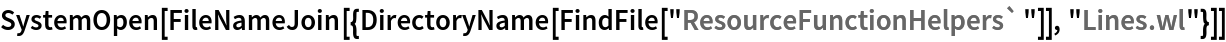Function Repository Resource:

# NormalLineThrough

Compute properties of the line normal (perpendicular) to a given line and passing through a given point

Contributed by: Wolfram|Alpha Math Team
 ResourceFunction["NormalLineThrough"][expr,{x,a},{y,b}] returns an Association of properties for the line passing through the point {x,y}={a,b} and normal to expr, a linear function in x. ResourceFunction["NormalLineThrough"][expr,{x,a},{y,b},prop] returns the normal line property prop.

## Details

Allowed values for prop are:
 "SlopeInterceptEquation" equation of the normal line in slope-intercept form "StandardFormEquation" equation of the normal line in standard form "PointSlopeEquation" equation of the normal line in point-slope form "Slope" slope of the normal line "HorizontalIntercept" horizontal intercept of the normal line "VerticalIntercept" vertical intercept of the normal line "Plot" plot of the normal line All Association of all allowed properties
If expr does not have head Equal, then expr is treated as an expression defining y in terms of x. In other words, ResourceFunction["NormalLineThrough"][expr,{x,a},y,] is equivalent to ResourceFunction["NormalLineThrough"][y==expr,{x,a},y,] if expr has a head other than Equal.

## Examples

### Basic Examples (2)

Get the point-slope equation of a line normal to y=3x/2+4 that passes through the point (x,y)=(1,-1):

 In:=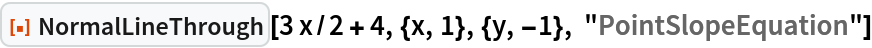Out=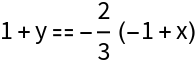Visualize this result:

 In:=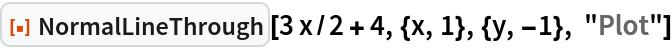Out=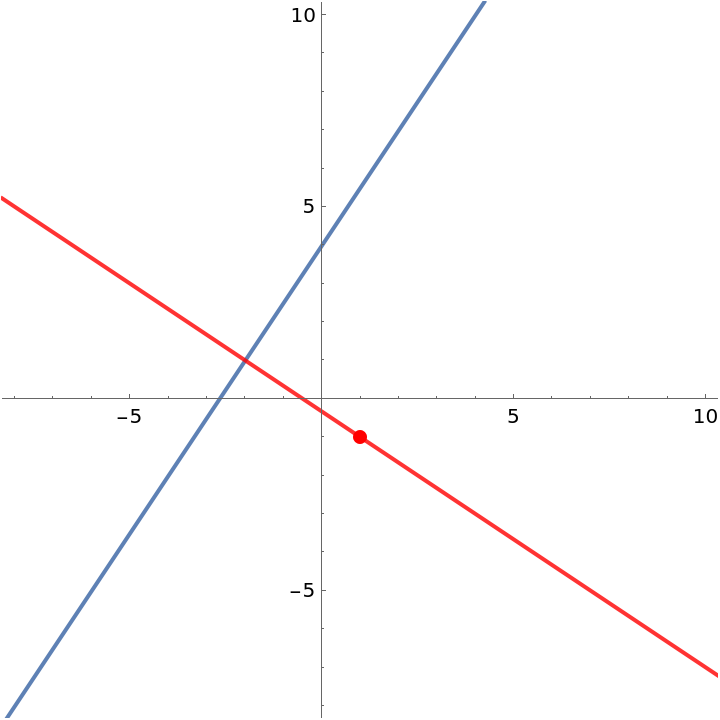Compute the slope of this line:

 In:=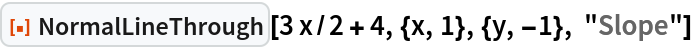Out=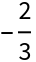Compute the horizontal intercept of this line:

 In:=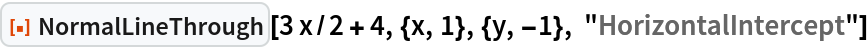Out=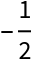Get the standard form equation of this line:

 In:=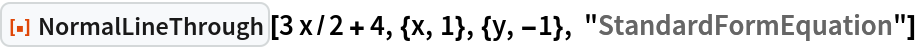Out=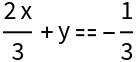Get an Association of properties of a line normal to y=-x/2+4 that passes through the point (x,y)=(3/5,6/7):

 In:=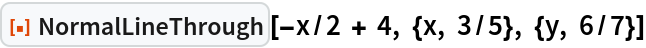Out=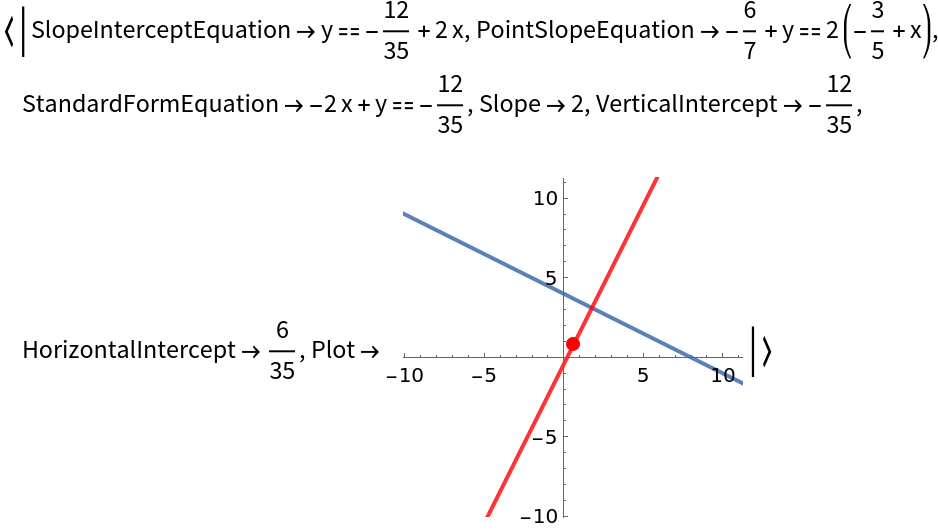Get just the slope-intercept equation of this line:

 In:=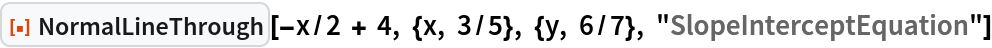Out=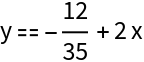### Possible Issues (1)

The first argument to NormalLineThrough must be a linear function of x:

 In:=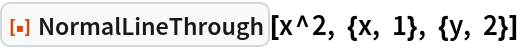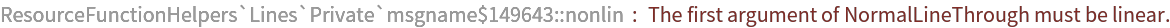Out=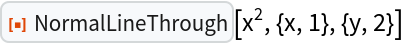## Publisher

Wolfram|Alpha Math Team

## Version History

• 2.0.0 – 23 March 2023
• 1.0.0 – 05 April 2022

## Author Notes

To view the full source code for NormalLineThrough, evaluate the following:

 In:=